# RS Aggarwal Solutions for Class 12 Maths Chapter 11: Applications of Derivatives Exercise 11B

Errors and Approximation are the concepts which are explained under this exercise. Students who are not able to obtain a clear idea about these concepts during class hours can revise them with the help of solutions PDF. For this purpose, a set of experienced teachers at BYJU’S design solutions as per the exam pattern of the current education board. RS Aggarwal Solutions Class 12 Maths Chapter 11 Applications of Derivatives Exercise 11B PDF links are available here for free download.

## RS Aggarwal Solutions for Class 12 Maths Chapter 11: Applications of Derivatives Exercise 11B Download PDF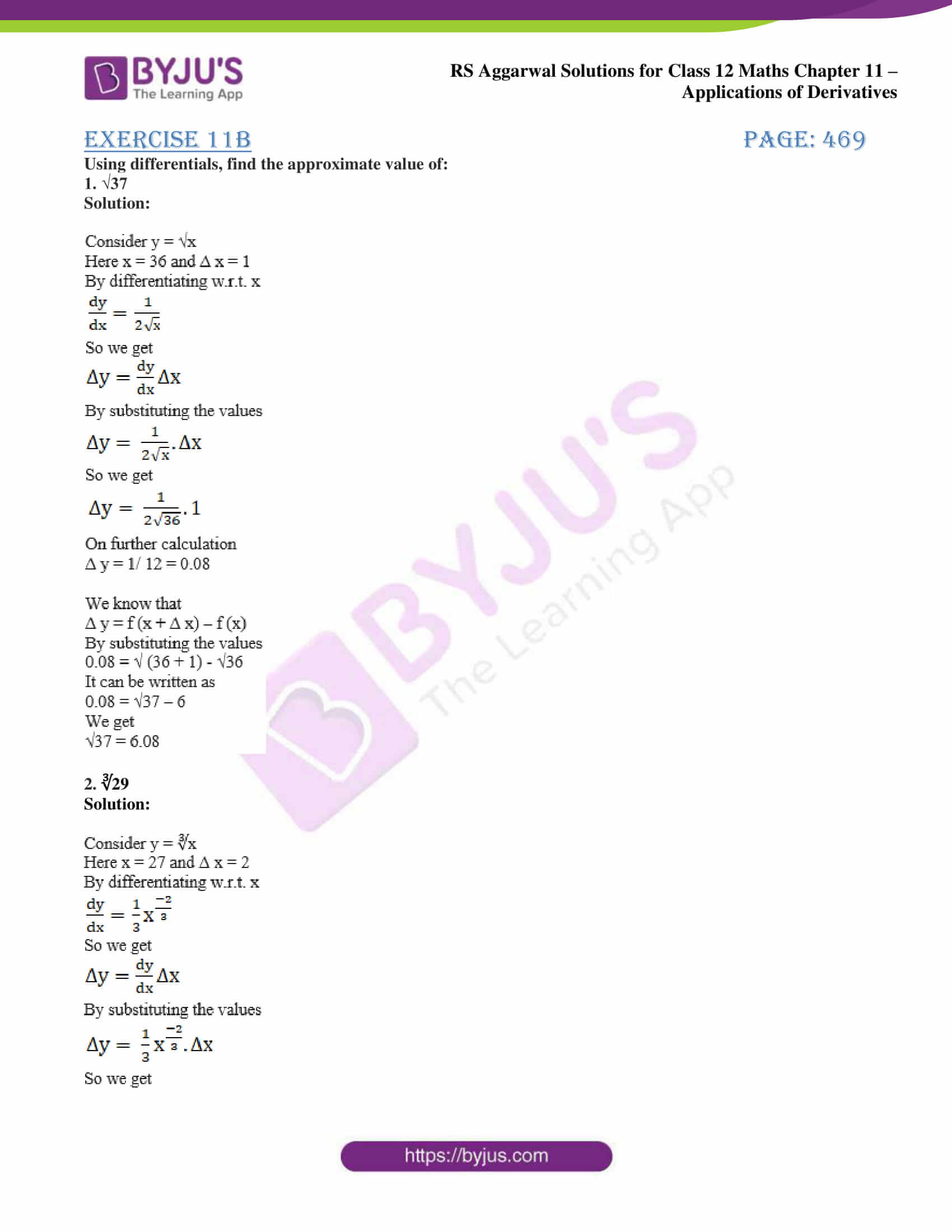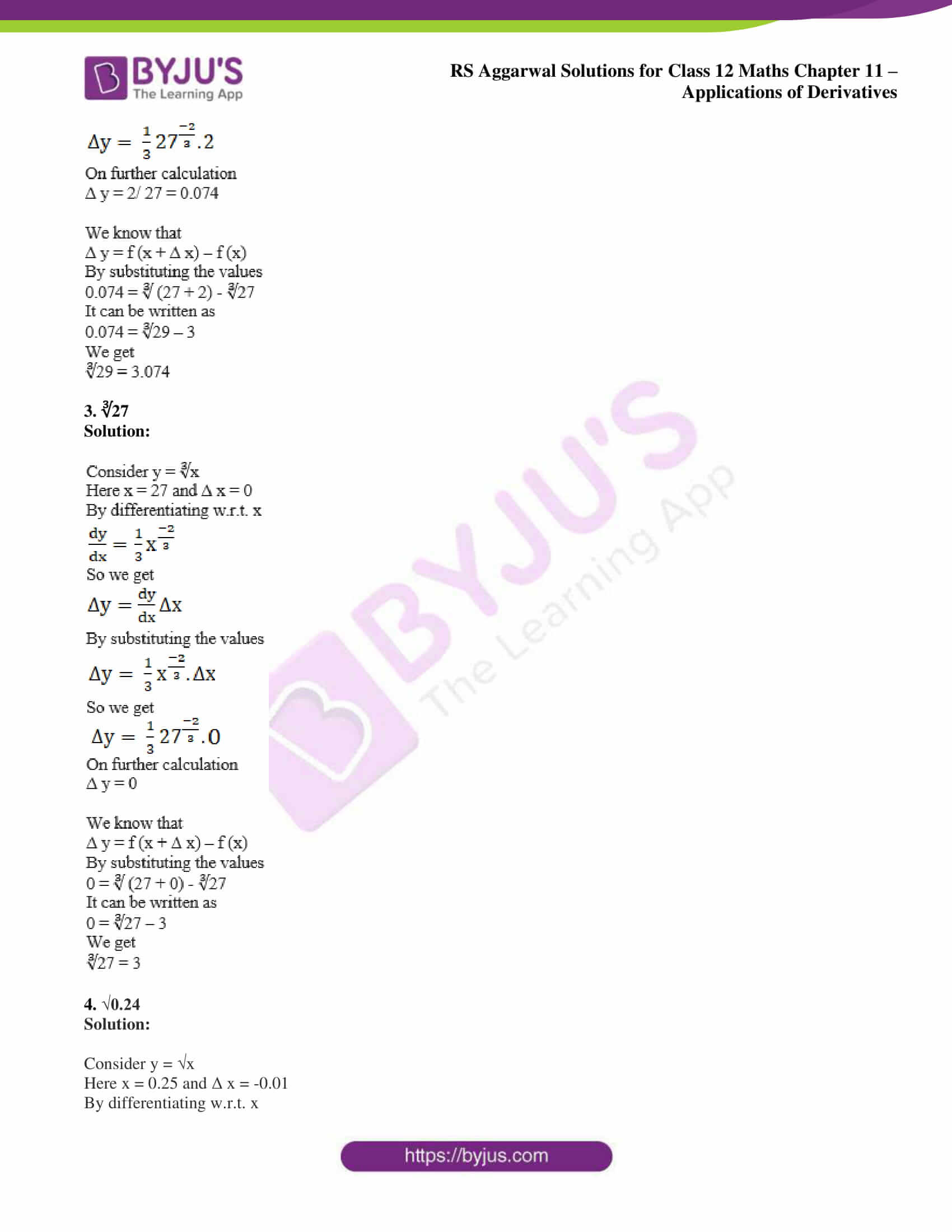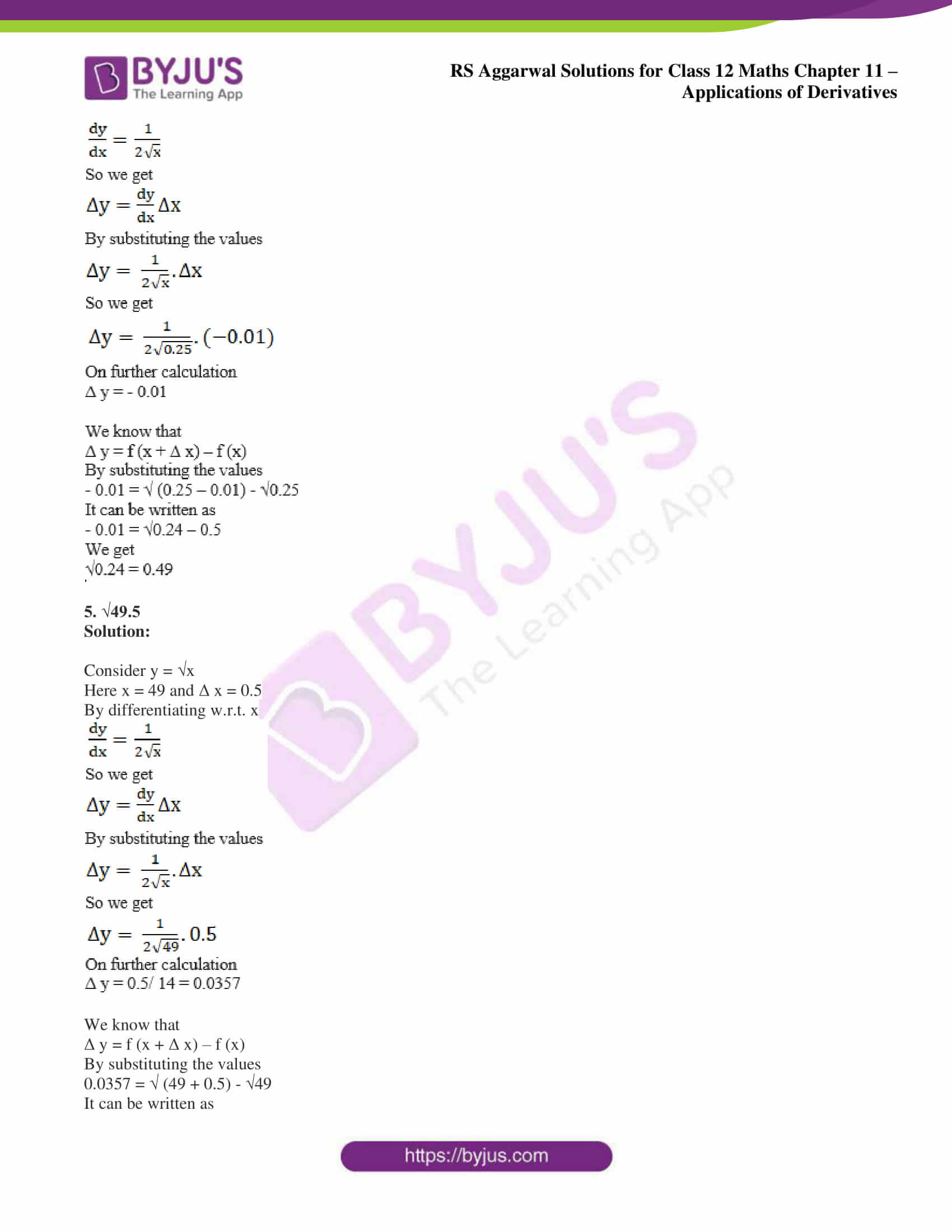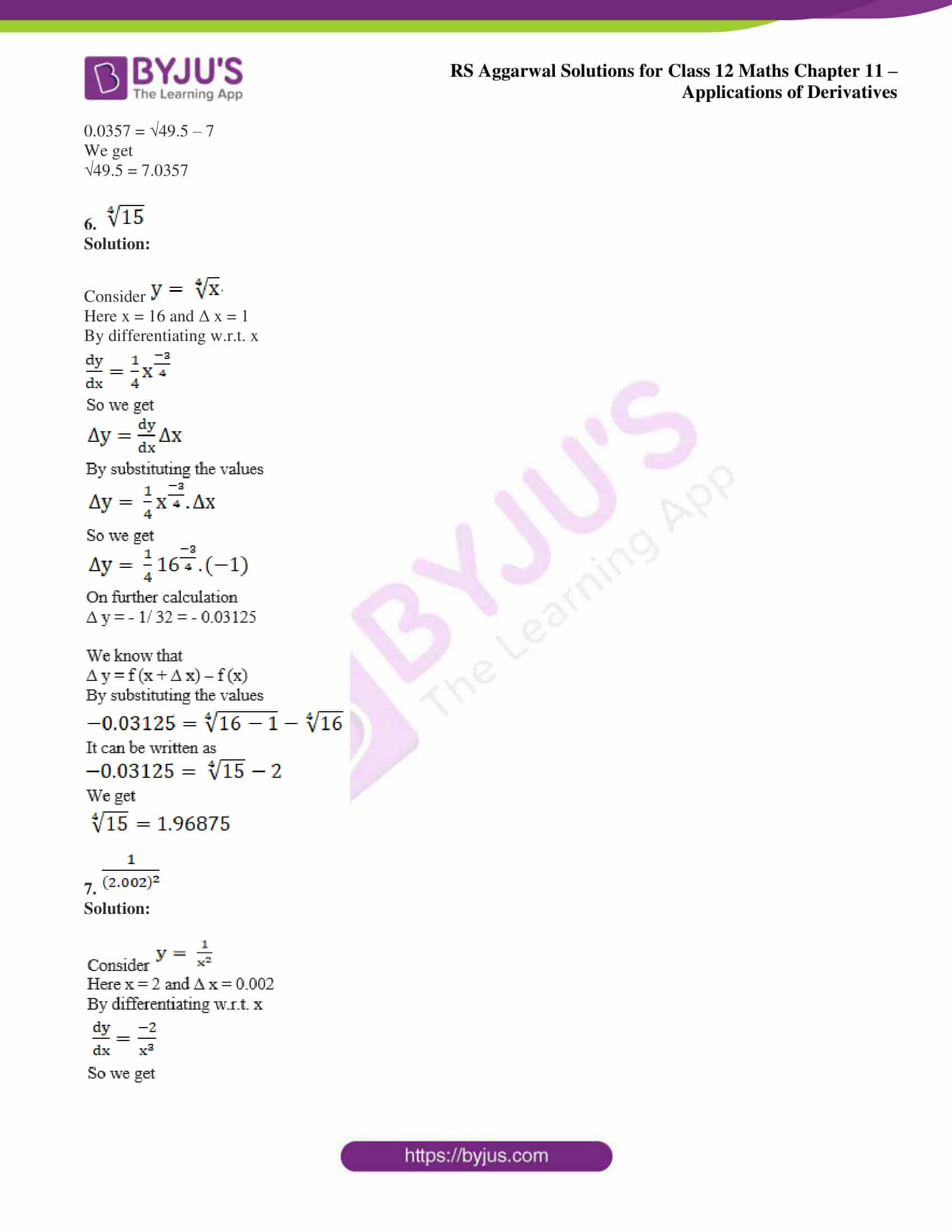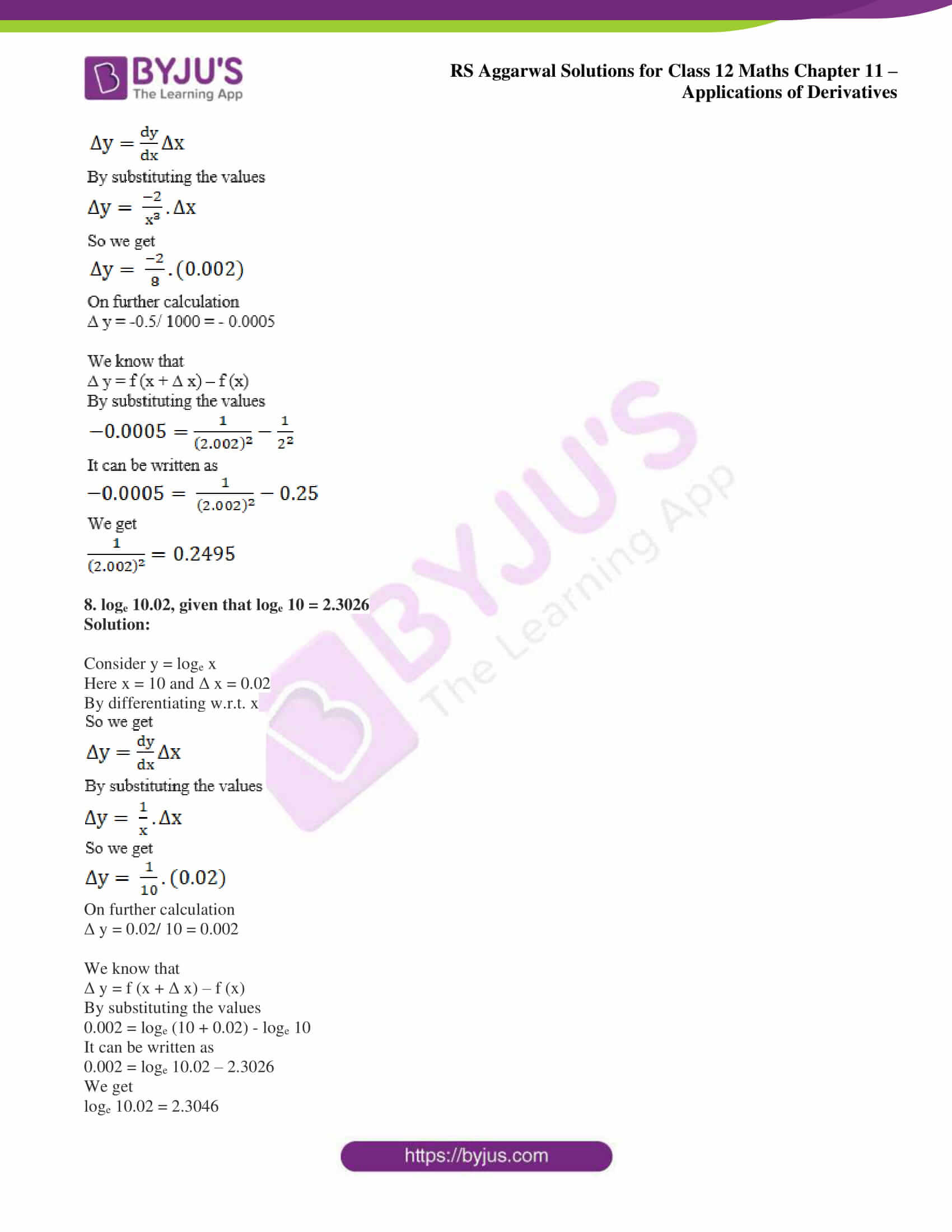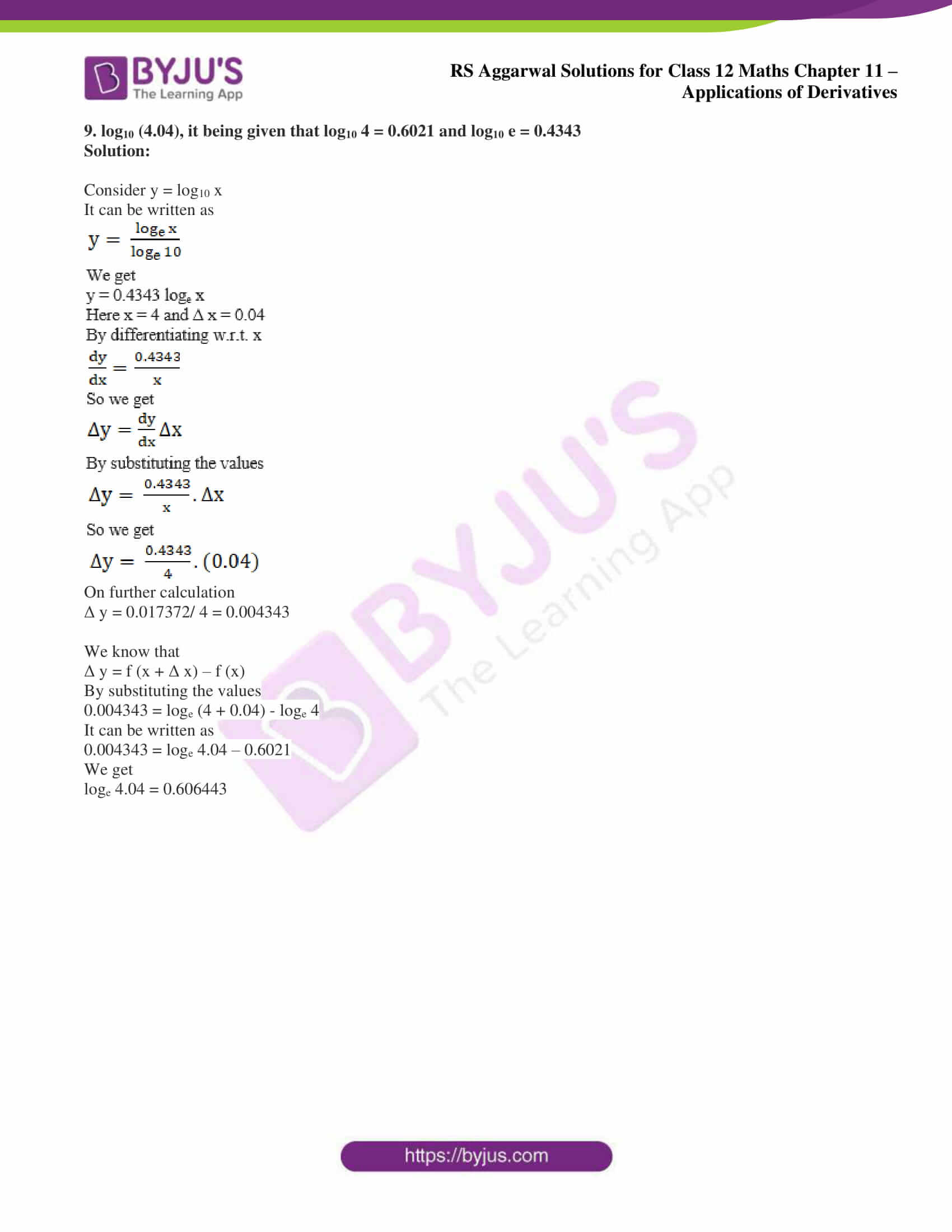### Access other exercise solutions of Class 12 Maths Chapter 11: Applications of Derivatives

Exercise 11A Solutions

Exercise 11C Solutions

Exercise 11D Solutions

Exercise 11E Solutions

Exercise 11F Solutions

Exercise 11G Solutions

Exercise 11H Solutions

### Access RS Aggarwal Solutions for Class 12 Maths Chapter 11: Applications of Derivatives Exercise 11B

Using differentials, find the approximate value of:

1. √37

Solution: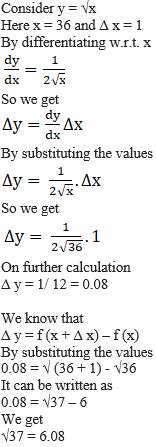2. ∛29

Solution: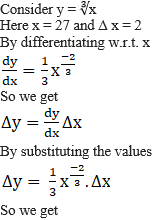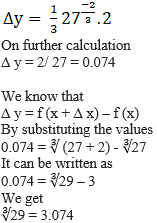3. ∛27

Solution: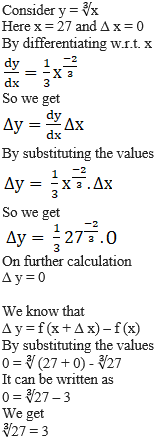4. √0.24

Solution:

Consider y = √x

Here x = 0.25 and Δ x = -0.01

By differentiating w.r.t. x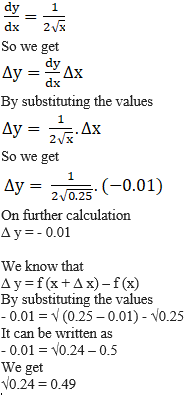5. √49.5

Solution:

Consider y = √x

Here x = 49 and Δ x = 0.5

By differentiating w.r.t. x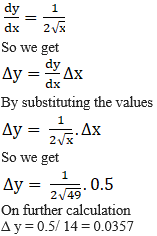We know that

Δ y = f (x + Δ x) – f (x)

By substituting the values

0.0357 = √ (49 + 0.5) – √49

It can be written as

0.0357 = √49.5 – 7

We get

√49.5 = 7.0357

6.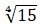Solution:

Consider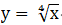Here x = 16 and Δ x = 1

By differentiating w.r.t. x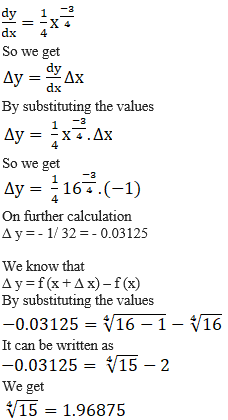7.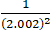Solution: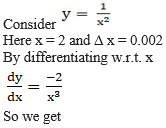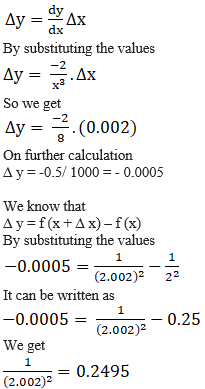8. loge 10.02, given that loge 10 = 2.3026

Solution:

Consider y = loge x

Here x = 10 and Δ x = 0.02

By differentiating w.r.t. x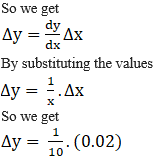On further calculation

Δ y = 0.02/ 10 = 0.002

We know that

Δ y = f (x + Δ x) – f (x)

By substituting the values

0.002 = loge (10 + 0.02) – loge 10

It can be written as

0.002 = loge 10.02 – 2.3026

We get

loge 10.02 = 2.3046

9. log10 (4.04), it being given that log10 4 = 0.6021 and log10 e = 0.4343

Solution:

Consider y = log10 x

It can be written as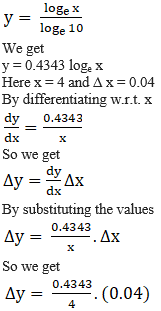On further calculation

Δ y = 0.017372/ 4 = 0.004343

We know that

Δ y = f (x + Δ x) – f (x)

By substituting the values

0.004343 = loge (4 + 0.04) – loge 4

It can be written as

0.004343 = loge 4.04 – 0.6021

We get

loge 4.04 = 0.606443